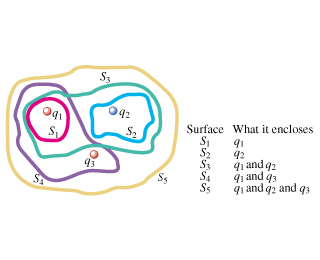# 042Surface What it encloses14241 and q4341 and q3S5Saq1 and q2 and q

Question
51 views

The three small spheres shown in the figure (Figure 1)carry charges q 1 = 3.70 nC , q 2 = -7.60 nC , and q 3 = 2.20 nC . Figure 1 of 1
Part A Find the net electric flux through the closed surface S 1 shown in cross-section in the figure.
Part B Find the net electric flux through the closed surface S 2 shown in cross-section in the figure.
Part C Find the net electric flux through the closed surface S 3 shown in cross-section in the figure.
Part D Find the net electric flux through the closed surface S 4 shown in cross-section in the figure.
Part E Find the net electric flux through the closed surface S 5 shown in cross-section in the figure.
Part F Do your answers to parts A through E depend on how the charge is distributed over each small sphere?help_outlineImage Transcriptionclose042 Surface What it encloses 1 42 41 and q 43 41 and q3 S5 Sa q1 and q2 and q fullscreen
check_circle

Step 1

Hey, since there are multiple subparts posted, we will answer first three questions. If you want any specific question to be answered then please submit that question only or specify the question number in your message

Step 2

The electric flux through any closed surface can defined as

Step 3

(a) The net electric flux through the...

### Want to see the full answer?

See Solution

#### Want to see this answer and more?

Solutions are written by subject experts who are available 24/7. Questions are typically answered within 1 hour.*

See Solution
*Response times may vary by subject and question.
Tagged in

### Electric Charges and Fields